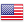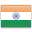Grade "A+" Accredited by NAAC with a CGPA of 3.46
Grade "A+" Accredited by NAAC with a CGPA of 3.46

# Financial Time Series Econometrics

Course ID
MDF 605
Level
Program
BMS
Semester
Sixth
Credits
6.0
Paper Type
DSE – Finance
Method
Lecture & Tutorial

## Unique Paper Code: Update Awaited

This course provides a comprehensive introduction to financial econometric concepts and techniques. It covers financial time Series econometrics, regression models with cross- sectional financial data, Asset price volatility models, simultaneous-equation models in financial time series, and economic forecasting.

## Learning Outcomes:

At the end of the course, students should be able to:

• Understanding of financial econometric concepts and techniques
• Interpretation of regression models with cross-sectional financial data
• Understanding of Asset price volatility models
• Understanding of simultaneous-equation models in financial time series, and economic forecasting.

## Course Contents

Unit I
Unit II
Unit III
Unit IV

Unit I (3 Weeks)

Financial time Series econometrics: Stationary stochastic processes, nonstationary Stochastic Processes, unit root stochastic processes, trend Stationary and difference Stationary stochastic processes. Tests of stationarity- graphical analysis and autocorrelation function (ACF) and correlogram statistical significance of autocorrelation coefficients. The unit root test – the augmented dickey-fuller (ADF) test. Transforming nonstationary financial time series – difference stationary processes and trend-Stationary process. Cointegration: regression of a unit root financial time series on another unit root financial time series, testing for cointegration and Cointegration and Error Correction Mechanism (ECM).

References:

Gujarati, N. Damodar. Basic Econometrics. New Delhi: McGraw Hill. [Chapter 21and 22]

Gujarati, N. Damodar. Econometrics by Examples. New Delhi: McGraw Hill.[Chapter 13 and 14]

Unit II (3 Weeks)

Regression models with cross-sectional financial data: The logit and Probit models, multinomial regression models, Ordinal regression models, and Limited dependent variable regression models.

References:

Gujarati, N. Damodar. Basic Econometrics. New Delhi: McGraw Hill. [Chapter 15-17]

Gujarati, N. Damodar. Econometrics by Examples. New Delhi: McGraw Hill.[Chapter 8-11]

Unit III (3 Weeks)

Asset price volatility: The ARCH and GARCH models. Extensions of the ARCH model. Simultaneous-equation models in financial time series: The nature of simultaneous-equation models, simultaneous-equation models, simultaneous-equation bias, inconsistency of OLS estimators. A test of simultaneity, tests for exogeneity. Simultaneous-Equation Methods – approaches to estimation, recursive models and ordinary least squares, estimation of a just identified equation, the method of indirect least squares (ILS), estimation of an overidentified equation: the method of two-stage least squares (2SLS)

References:

Gujarati, N. Damodar. Basic Econometrics. New Delhi: McGraw Hill. [ Chapter 17 – 20]

Gujarati, N. Damodar. Econometrics by Examples. New Delhi: McGraw Hill. [13 and 15]

Unit IV (3 Weeks)

Economic forecasting: Forecasting with regression models. The Box–Jenkins methodology: ARIMA modeling. An ARMA model of companies daily closing prices. Vector autoregression (VAR), Testing causality using VAR: The Granger causality test

References:

Gujarati, N. Damodar. Basic Econometrics. New Delhi: McGraw Hill. [Chapter 22]

Gujarati, N. Damodar. Econometrics by Examples. New Delhi: McGraw Hill. [Chapter 16]

#### Text Books

Christopher Dougherty. Introductory Econometrics. Oxford University Press.
Gujarati, N. Damodar. Basic Econometrics. New Delhi: McGraw Hill.
Gujarati, N. Damodar. Econometrics by Examples. New Delhi: McGraw Hill.

Pindyck, Robert S. and Daniel L. Rubinfeld Econometric Models and Economic Forecasts. Singapore: McGraw Hill.
Ramanathan, Ramu (2002). Introductory Econometrics with Applications (5th ed.). Thomson South Western

#### Teaching Learning Process

This course will be taught using a mix of the following tools:
1. Relevant Case studies
2. Explanation of econometric tools using software like R and Stata.
3. Relevant and important research articles from academic linked journals in the domain of Management such as Harvard Business Review,

#### Assessment Methods

The total assessment of the course is for 100 marks and would be split as follows:
D. Semester end exam = 75 marks
E. Attendance = 5 marks
F. Internal = 20 marks (5 – class participation; 5 – term paper; 10 – class presentation)

#### Keywords

Financial Econometric, regression models with cross-sectional financial data, Asset price volatility, simultaneous-equation, economic forecasting.

Disclaimer: Details on this page are subject to change as per University of Delhi guidelines. For latest update in this regard please refer to the University of Delhi website here.Englishहिन्दी